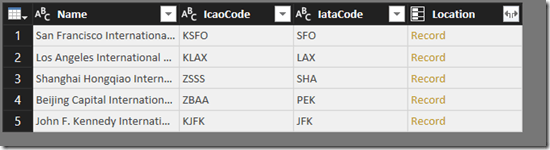# A Function To Generate The M Code For A Table Type

This is going to sound obscure, and it is, but recently I’ve been using the #table() intrinsic function in M a lot – specifically the version that takes a table type as its first parameter (as I describe here) – and because it’s a bit of a pain to have to write the M code for a table type by hand, I’ve written an M function that takes a table and returns the text for the M code that is needed to define a table type. Here it is:

```(InputTable as table) as text =>
let
Source =
Table.Schema(InputTable),
SortRows =
Table.Sort(
Source,
{{"Position", Order.Ascending}}),
RemoveColumns =
Table.SelectColumns(
SortRows,
{"Name", "TypeName"}),
RemoveColumns,
"TypeNames",
each
Expression.Identifier([Name]) & " = " & [TypeName]),
Output =
"[" & Text.Combine(AddCustom[TypeNames], ", ") & "]"
in
Output
```

Nothing complex here, but now I’ve posted this I know that in the future I’ll be able to Google for it when I’m working onsite with a customer and I need it!

To give you an idea of how it works, take the table that is returned by the following M expression, which calls the public TripPin OData web service:

```OData.Feed(
"https://services.odata.org/TripPinRESTierService/Airports",
null,
[Implementation="2.0"])
```Passing this table to the function above returns the following text, the M code for a record that lists the names of the columns in this table and their data types, suitable for use with #table:

```[Name = Text.Type, IcaoCode = Text.Type,
IataCode = Text.Type, Location = Record.Type]
```

### 5 responses

1.Francis Blake says:

Just what I needed, and it works brilliantly! thanks for this.T
A few things you might want to consider:
1. It took me an incredible amount of googling to land here (I had almost given up). It wouldn’t be a problem if, as underlined by Dale Hohm* two years ago, the useful info on M wasn’t still made of bitty tips (of which this page is) scattered around the internet.
2. Even though I had a function to create the string for a row type (which is what M is expecting after the directive “type table”), I still had to work out how to make your trick work. As it is, a novice M user would not stand a chance of using it. Please consider adding a full example.
3. For some reason TypeName doesn’t work for me. PQ complains that Number.Type or Text.Type “are not valid in this context” when I evaluate the expression. However, the trick works well with the Kind column of the schema.

Here is what the full code looks like for me:

let
fTableType = (InputTable as table) as type =>
let
Source = Table.Schema (InputTable)
,SortRows = Table.Sort (Source, {{“Position”, Order.Ascending}})
each Expression.Identifier ([Name]) & ” = ” & [Kind])
,TypeString = “type table [” & Text.Combine(AddCustom[TypeNames], “, “) & “]”
,Output = Expression.Evaluate (TypeString)
in
Output
// Main body
,ExistingTable = #table (type table [Column1 = number, Column2 = text], {})
,Table = #table (fTableType(ExistingTable), {{1, “A”}, {2,”B”}})
in
Table

Note 1: I put the comma at the beginning of a line rather than the end; it is easier to spot when one is missing.
Note 2: Given the small size of the schema table, I didn’t think removing columns was a useful addition to the code, so for simplicity I removed that step. Hopefully I am not missing something important and arcane.

2.Alexander Kraijenoord says:

Thanks for this code!
I adjusted it, so the Table.ExpandTableColumn will keep the assigned column types after expansion

The table that I’m expanding is stored in a column called #”Removed Other Columns”[FirstRow]

and from there on the code runs like this:

TableStructure = let
InputTable = List.First(#”Removed Other Columns”[FirstRow]),
Source = Table.Schema(InputTable),
SortRows = Table.Sort(Source,{{“Position”, Order.Ascending}}),
RemoveColumns = Table.SelectColumns(SortRows,{“Name”, “Kind”}),
each Expression.Identifier([Name]) & ” = ” & [Kind]),
Output =
“[” & Text.Combine(AddCustom[TypeNames], “, “) & “]”
in
Output,
#”Expanded FirstRow” = Table.ExpandTableColumn(#”Removed Other Columns”, “FirstRow”, Table.ColumnNames(List.First(#”Removed Other Columns”[FirstRow]))),
#”Re-apply TableStructure” = let
TableSchema = Expression.Evaluate(“#table (type table ” & TableStructure &”, {})”),
Records = Table.ToRecords(#”Expanded FirstRow”),
Result = Table.InsertRows(TableSchema, 0, Records)
in Result
in
#”Re-apply TableStructure”

3.nickburns42 says:

This was the missing piece I was looking for to quickly add columns to a table. I’ve come up with 5 different methods to quickly add columns to a table.

//Named Colmuns, no Data Types
AddNewColumns = PreviousStep & #table({“Number Column”, “Text Column”, “Date Column”}, {})

//Name Columns with different Data Types
AddNewColumns = PreviousStep & #table( type table
[
#”Number Column”=number,
#”Text Column”=text,
#”Date Column”=date
], {})

//Generic Columns no Data Types
AddNewColumns = PreviousStep & #table(List.Transform({1..5}, each “Undefined” & Text.From(_)), {})

//Generic Columns with equivalent Data Types (thanks to this post)
AddNewColumns = PreviousStep & #table(Expression.Evaluate(“type table [” & Text.Combine(List.Transform({1..5}, each “Undefined” & Text.From(_) & ” = number”), “, “) & “]”), {})

//Generic Columns using List.Generate with equivalent Data Types
Add_Multiple_Columns = List.Accumulate({1..5}, PreviousStep, (state, current) => Table.AddColumn(state, “UserDefined” & Text.From(current), each null, type number))

This site uses Akismet to reduce spam. Learn how your comment data is processed.